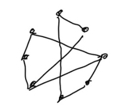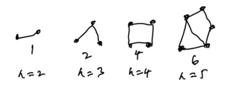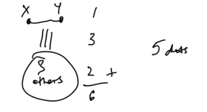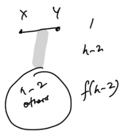## Dots and Lines

Visible to anyone in the world
Edited by Richard Walker, Monday, 7 Dec 2020, 22:11

I'm a big doodler. Last night I drew some dots in a rough octagon and started drawing lines joining pairs of dots.Then I wondered how many lines I could draw before I formed a triangle. I don't mean a triangle formed by three lines (although that's an interesting question too), but one with dots at its three corners.

With any problem where you have a series it's always a good idea to look at the small cases. Sometimes it can be misleading though. Still I drew some little sketchesIt's easy to be sure the count is right for n = 2, 3 and 4, but even by 5 it gets less obvious. Is there a general formula? I wondered if this was a novel puzzle (and hoped it was); sadly it turns out no, it was investigated by Mantel in 1907. There are various proofs of the result Mantel found, but they are more or less technical and not intuitive. So I wondered if I could present the basic idea of one proof in a more accessible way.With 5 dots consider a pair x and y that are joined by a line. That leaves 3 others and if we think about any one of these, let's call it z, we see x and y cannot both be joined to z. Otherwise we would have a triangle! So the maximum of lines from either x and y to the other dots is 3. Finally focusing on the 3 other dots, the maxiumum number of lines possible amongst these is 2, otherwise we would have a triangle.

Adding this all up as shown the greatest possible number of lines is 1 + 3 + 2 = 6.

Let's see if we can generalise this idea. Suppose we have n dots and the maximum number of lines that can be drawn without forming a triangle is f(n).Following the same argument as before, if we are to avoid a triangle, we can have one line joining x to y, a maximum of n - 2 lines joining either x or y to one of the remaining n - 2 dots, and f(n - 2) lines amongst tos n - 2 others. So

f(n) = 1 + n - 2 + f(n - 2) = n - 1 + f(n - 2)

So we can immediate calculate further values

f(6) = 6 - 1 + f(6 - 2) = 5 + f(4) = 5 + 4 = 9

f(7) = 7 - 1 + f(7 - 2) = 6 + f(5) = 6 + 6 = 12

f(8) = 8 - 1 + f(8 - 2) = 7 + f(6) =  7 + 9 = 16

So now we have (for completeness we include n = 1 with 0 lines)

0, 1, 2, 4, 6, 9, 12, 16

Can you spot a pattern?

Share post

This blog might contain posts that are only visible to logged-in users, or where only logged-in users can comment. If you have an account on the system, please log in for full access.

Total visits to this blog: 1249787